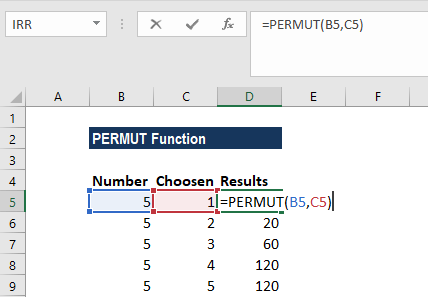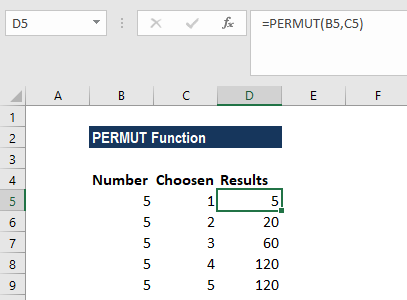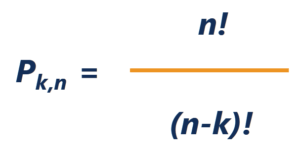# PERMUT Function

Calculates the number of permutations of a specified number of objects from a given set of objects

## What is the PERMUT Function?

The PERMUT Function is categorized under Excel FINANCIAL functions. The function will calculate the number of permutations of a specified number of objects from a given set of objects.

In financial analysis, PERMUT can be useful in calculating different possible permutations. So, it will help in rationally evaluating situations and making the correct choices on behalf of shareholders or clients.

### Formula

=PERMUT(number, number_chosen)

The PERMUT function uses the following arguments:

1. Number (required argument) – This is an integer that describes the number of objects.
2. Number_chosen (required argument) – An integer that describes the number of objects in each permutation.

### How to use the PERMUT Function in Excel?

As a worksheet function, the PERMUT function can be entered as part of a formula in a cell of a worksheet. To understand the uses of the function, let us consider an example:

#### Example 1

Let’s calculate the number of permutations of six objects selected from differently sized sets:

NumberChosenResultNotes
5155 possible permutations in group 1
522020 possible permutations in group 1
536060 possible permutations in group 1
54120120 possible permutations in group 1
55120120 possible permutations in group 1

We used the formula below:We get the results below:The number of permutations for a given number of objects is the number of combinations in each possible order.

Note that permutations differ from combinations in that, for a permutation, the order of the objects matters, but in a combination, the order does not matter.

The number of possible permutations is given by the formula:Where:

• k is the number of objects chosen
• n is the number of possible objects

### A few notes about the PERMUT Function:

1. #NUM! error – Occurs if either:
1. The given number is less than 0.
2. The given argument number_chosen is less than 0.
2. #VALUE! error – Occurs if one or both of the given arguments is non-numeric.
3. Arguments that contain decimal values are truncated to integers.
4. A combination is any a group of items in any order. If the order is significant, we should use the PERMUT function. If the order is not significant, we can use the COMBIN function.

Thanks for reading CFI’s guide to important Excel functions! By taking the time to learn and master these functions, you’ll significantly speed up your financial analysis. To learn more, check out these additional CFI resources:

• Excel Functions for Finance